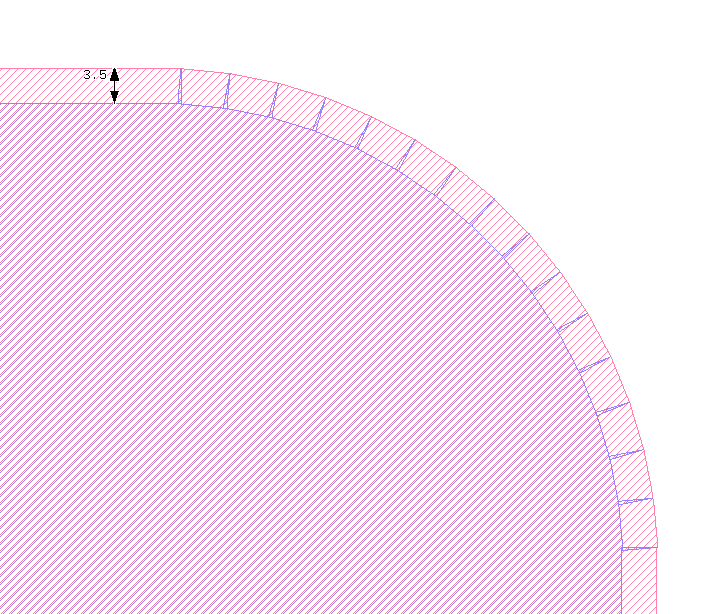# Python DPolygon.sized() produces little triangles

edited February 2

I am trying to script a shape with rounded corners. When I use the `sized()` function on a `DPolygon` I get little darts on the rounded corners. In the example below I resize the red shape by -3.5 um to get the blue shape. A similar effect also happens with a positive sizing on an interior corner. It is also not specific to rounded corners. I get something very similar with 90 degree corners.

This does not occur when using the GUI: Menu:Edit:Selection:Size Shapes.

Is there a way to get the same behavior as the GUI in a Python script? It seems like it might be related to having a `DPolygon` instead of a `DSimplePolygon`. But I cannot figure out how to create a `DSimplePolygon` from a `DPolygon` in Python.

I am using KLayout version 0.27.11 on Windows.
Suggestions are appreciated!• Hello yanoff,

I got the same result as you mentioned, but `Region` is ok. Not sure it works to you?

Vincent

• I'm wondering whether this is "algorithmic" (and a bug) due to the
high vertex count and long shrink dimension, causing "spurious
projection" as the vertices might cross over each other when projected
orthogonal to their flat? Or the single vertex becoming two, as the
two flats' projections will differ?

What happens (say) with a shrink-distance that is much less than the
vertex-vertex distance? Just by way of debug. What happens if it's
N=36 vertices instead of N=64 (or whatever)?

• Hello,

no that's not a bug. Polygon#sized is not intended to do a full job. Instead you need to "merge" the polygon to remove the triangles (see https://www.klayout.de/doc-qt5/code/class_DPolygon.html#m_size).

Background is: the size polygon in the general case will create 0 to n polygons - a negative sizing may render smaller, disconnected or even no polygon at all. So there is no polygon-to-polygon size function. The "Region" class is a collection of polygons, hence "sized" works here.

There is a problem with "DPolygon": you first need to convert it to a integer-unit polygon before you can use it in the edge processor (as suggested in the documentation) or inside Region. If you have a minimum grid (database unit), you can use this transformation to create a Polygon:

``````dbu = 0.001   # 1 nm resolution
dpoly = ...   # your DPolygon object

# convert the DPolygon to Polygon
poly = RBA::CplxTrans::new(dbu).inverted * dpoly

# do something with the polygon, e.g.
region = RBA::Region::new
region.insert(poly)
# perform the size, assuming it will always produce a single polygon (CAUTION: this may not be true, see above)
poly = region.sized(size_value)

# convert it back to DPolygon
dpoly = RBA::CplxTrans::new(dbu) * poly
``````

Matthias

The problem was driven by trying to size() a DPolygon. The result was a shape with dimensions in nanometers instead of micrometers. I think another part of the problem was points snapping to the 1 nm grid points.

I did find the method DPolygon.to_itype(dbu) to convert from DPolygon to Polygon. That seems more natural to me.

• edited February 16

@yanoff Be careful: `DPolygon.to_itype` does not include the scaling of micrometers to database units. It will simply round to integers.

It's only a convention, but `DPolygon` often represents a polygon in floating-point micrometer units while `Polygon` uses integer units which give you micrometers when multiplied by the database unit. Transformation between these spaces is done best using the `CplxTrans` objects as shown above.

Matthias

• Both `to_itype()` and `to_dtype()` take an optional dbu argument. If this is provided, they do perform the scaling, don't they?

• Yes, that is supposed to provide the database unit you intend to have in integer space.

So basically

``````DPolygon = Polygon * dbu

Polygon = DPolygon * (1/dbu)
``````

Matthias